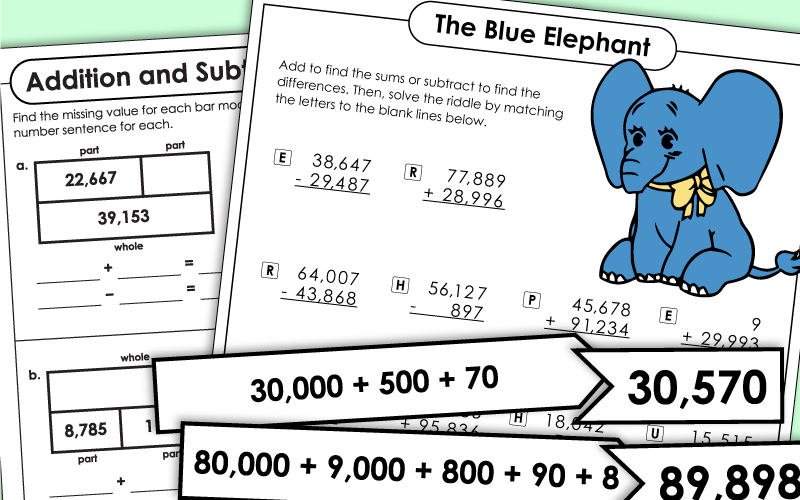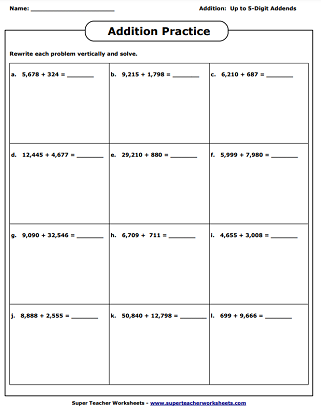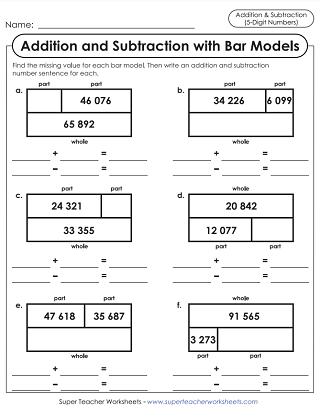These addition problems are written on graph paper squares to help students better see the place value column alignment.
Solve the addition problems in the boxes. This worksheet has space for students to rewrite problems vertically (if needed) and show their work.
These two addition problems have been solved incorrectly. Can you find the errors? Tell how each problem could be solved correctly.
Practice a variety of 5-digit addition skills with this worksheet.
Add each pair of addends (up to 5 digits). Then sort them into two categories: odd sums and even sums.
Choose a number from each of the two circles and add them together. Choose six different pairs of numbers to add.
Solve the addition problems. Place the sums into the correct places on the crossword puzzle.

## Expanded Form

Match each "expanded form" number with the "standard form" number. This is a cut-and-play activity.
Write each number in expanded form. This airplane-themed activity includes 6 problems and 2 word problems.

Solve 5-digit addition and subtraction problems. Then use the answers to decode the answer to a funny riddle: What should you do if you find a blue elephant?
Students use their addition and subtraction skills to complete six part-part-whole bar models.
Add and subtract using bar models. Find the missing number for each model illustration. This worksheet has space for students to show their work.

Create your own addition worksheets with randomized numbers. You choose the number of digits in each addend.

Subtraction Worksheets

This page has printable worksheets for teaching basic subtraction facts, multi-digit subtraction, subtracting fractions, and decimal subtraction.

## Sample Worksheet ImagesMy Account
Site Information